### Applicable Tests and Sample Size Computation

The SEQDESIGN procedure assumes that the data are from a multivariate normal distribution and the sequence of the standardized test statistics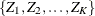have the following canonical joint distribution:

•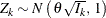•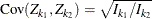,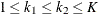where K is the total number of stages and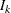is the information available at stage k.

If the data are not from a normal distribution such as binomial distribution, then it is assumed that the test statistic is computed from a large sample such that the statistic has an approximately normal distribution.

In a clinical trial, the sample size required depends on the Type I error probability, reference improvement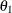, power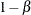, and variance of the response variable. Given a null hypothesis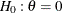with an upper alternative hypothesis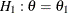, the information required for a fixed-sample test is given by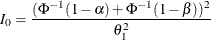where the parameterdepends on the test specified in the clinical trial. For example, if you are comparing two binomial populations, then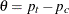is the difference between two proportions if the proportion difference statistic is used, and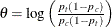, the log odds ratio for the two proportions if the log odds ratio statistic is used.

If the maximum likelihood estimate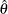from the likelihood function can be derived, then the asymptotic variance foris Var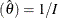, where I is Fisher’s information for.

The resulting statisticcorresponds to the MLE scale as specified in the BOUNDARYSCALE=MLE option in the PROC SEQDESIGN statement,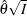corresponds to the standardized Z scale (BOUNDARYSCALE=STDZ), and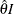corresponds to the score scale (BOUNDARYSCALE=SCORE).

Alternatively, if the score statistic is derived, it can also be used as the test statistic and its asymptotic variance is given by Fisher’s information.

For a group sequential trial, the maximum information
is derived in the SEQDESIGN procedure by using the specified,, and. With the maximum information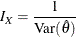the sample size required for a specified test statistic in the trial can be evaluated or estimated from the known or estimated variance of the response variable. Note that different designs might produce different maximum information levels for the same hypothesis, and this in turn might require a different number of observations for the trial.

With a specified test statistic, the required sample sizes at the stages can be computed. These tests include commonly used tests for normal means, binomial proportions, and survival distributions. See the section Sample Size Computation in The SEQDESIGN Procedure for a description of these tests.